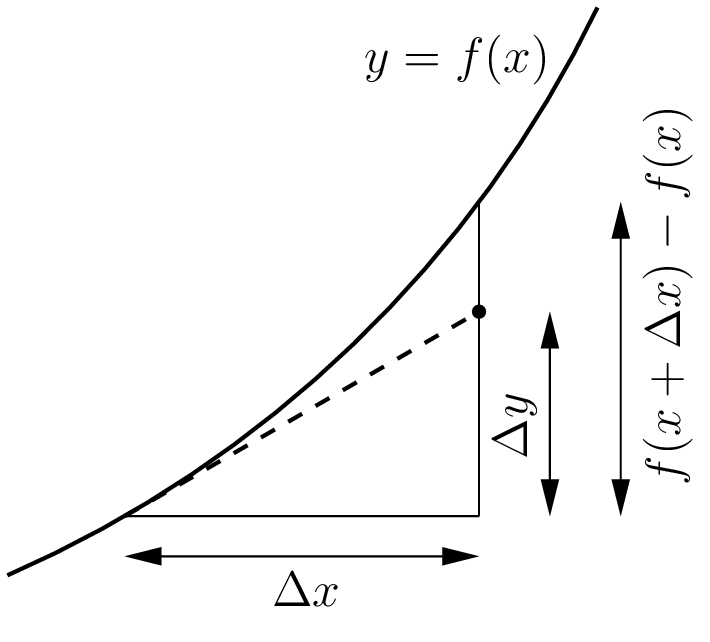## Section7.4DifferentialsFigure 7.4.1. Linear approximation using the tangent line.

The tangent line to the graph of $$y=f(x)$$ at the point ($$x_0\text{,}$$$$y_0$$) is given by

\begin{equation} y-y_0 = m \left( x-x_0 \right)\tag{7.4.1} \end{equation}

where the slope $$m$$ is of course just the derivative $$\frac{df}{dx} \big|_{x=x_0}\text{.}$$ It is tempting to rewrite the equation of the tangent line as

\begin{equation} \Delta y = \frac{df}{dx} \Delta x\tag{7.4.2} \end{equation}

which is also used for linear approximation in the form

\begin{equation} \Delta f = f(x+\Delta x)-f(x) \approx \frac{df}{dx} \Delta x\tag{7.4.3} \end{equation}

as shown in Figure 7.4.1. Regarding $$\Delta x$$ as small, we rewrite (7.4.3) in the form

\begin{equation} df = \frac{df}{dx} \,dx\tag{7.4.4} \end{equation}

where the differential $$df$$ can be interpreted as the corresponding small change in $$f\text{.}$$

The intuitive idea behind differentials is to consider the small quantities “$$dy$$” and “$$dx$$” separately, with the derivative $$\frac{dy}{dx}$$ denoting their relative rate of change. So rather than either of the expressions used by Newton or Leibniz (see Section 7.3), if $$y=x^2$$ we write

\begin{equation} dy = 2x\,dx .\tag{7.4.5} \end{equation}

More generally, if $$y$$ is any function of $$x\text{,}$$ then the derivative $$\frac{dy}{dx}$$ relates the differentials $$dy$$ and $$dx$$ via

\begin{equation} dy = \frac{dy}{dx} dx .\tag{7.4.6} \end{equation}

You can safely think of (7.4.5) as a sufficiently small quantity, or as the numerator of Leibniz notation, or as shorthand for a limit argument, or in terms of differential forms, or nonstandard analysis, or ...; it doesn't matter, they all give the same equations.